Subsections

# Ideal Gas

## Main Assumption and Partition Function

Definition:
Ideal Gas is the system where interaction is absent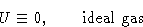Configuration Integral:
This is factorizable!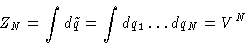Partition Function: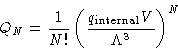## Thermodynamic Properties

### Stirling Formula

Let us calculate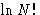for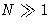: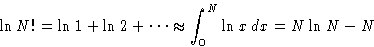### Free Energy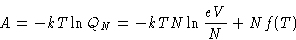with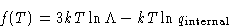### Pressure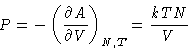Pressure of ideal gas does not depend on the nature of the gas!

### Entropy and Heat Capacity:

Entropy: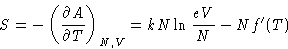(4)
or, substituting V by NkT/P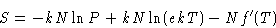(5)
Heat capacity: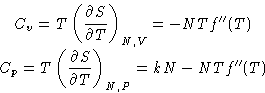We obtained:

Cp-Cv = kN

### Energy

 E = A+TS = Nf(T)-NTf'(T) (6)Suppose we have a container of the volume 2V with 2N particles, divided into two parts. We deleted the divider. What is the entropy change?
First Method:
Let us paint the particles in the left in white, and particle in the right in black. After we deleted the divider, white'' and black'' particles mix. From equation (4) the change of entropy for white particles'' is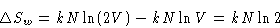For black particles''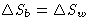. Total change of entropy is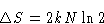(7)
Second Method:
Entropy is an extensive variable, so the entropy for 2N molecules in the volume 2V is twice the entropy for N molecules in the volume V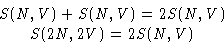and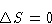(8)

What is wrong? Why we obtained different answers?

Solution:
The distinguishability is a quantum effect: it is either there, or not (particles cannot be a little bit different!). If they are identical, we cannot speak about entropy of white or black particles separately--and only (8) works. If they are not identical, only (7) works.
Consequence:
There is an entropy change when mixing different isotopes--even while the molecules are quite similar!Next: Quiz Up: Systems with Many Particles. Previous: Many-Particle Systems

© 1997 Boris Veytsman and Michael Kotelyanskii
Wed Sep 17 22:58:45 EDT 1997